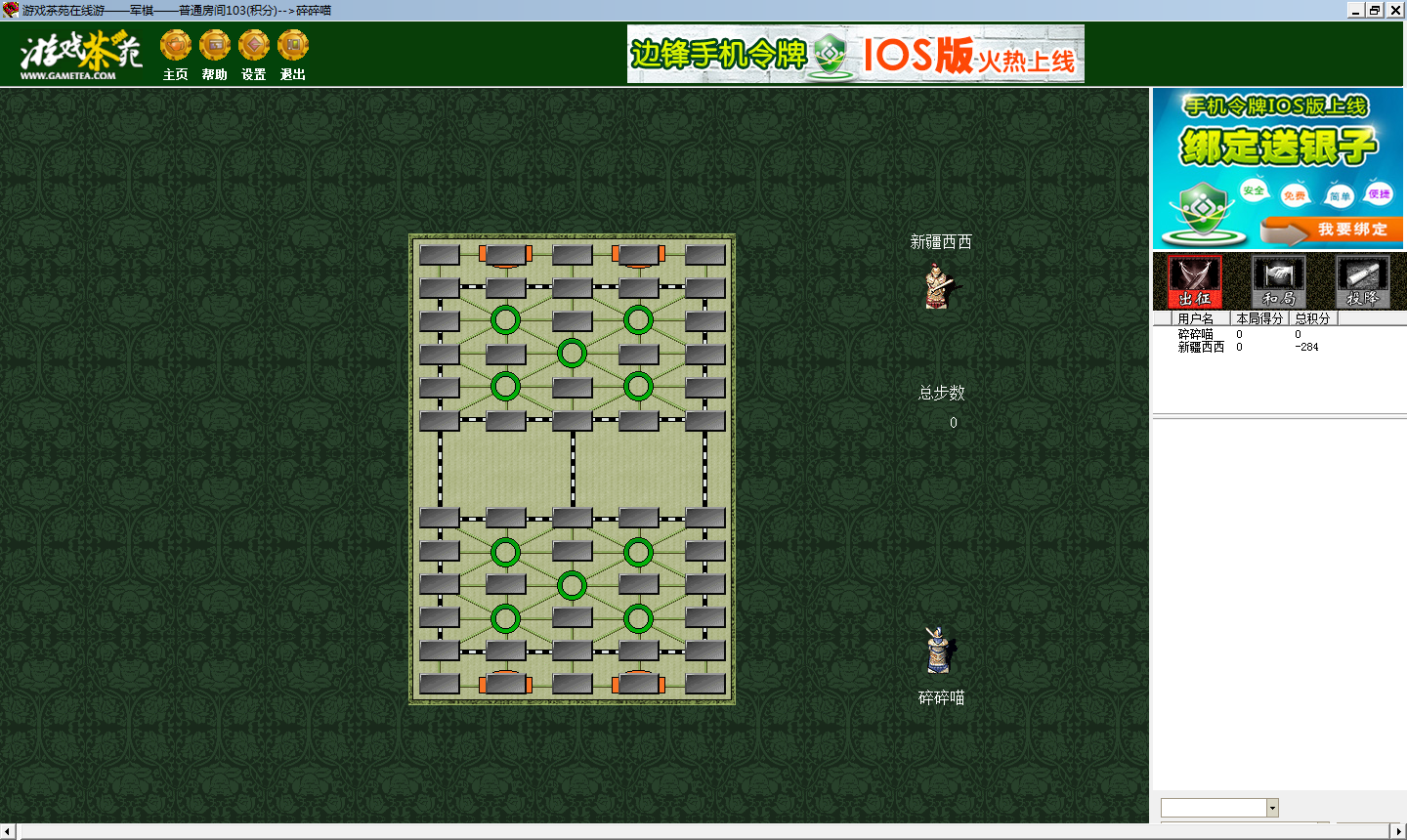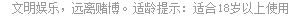# 军棋翻翻棋  当前版本: 2.0.0

#### 通过操作系统下载 - 最低系统版本 (安卓: 4.3; IOS: 8.0)>>### 游戏界面### 游戏规则

【积分规则】
 序列 等级 积分 序列 等级 积分 1 二等兵 <0 10 协参领 <= 5110 2 一等兵 <= 10 11 副参领 <= 10230 3 正兵 <= 30 12 正参领 <= 20470 4 下士 <= 70 13 协都统 <= 40950 5 中士 <= 150 14 副都统 <= 81910 6 上士 <= 310 15 正都统 <= 163830 7 协军校 <= 630 16 将军 <= 327670 8 副军校 <= 1270 17 大将军 <= 655350 9 正军校 <= 2550

## 客服中心

4007202233(9:00-21:00) 在线客服

## 常见问题